## Residue Theorem (Complex Analysis)

Given a complex function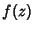, consider the Laurent Series(1)

Integrate term by term using a closed contour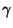encircling,(2)

The Cauchy Integral Theorem requires that the first and last terms vanish, so we have(3)

But we can evaluate this function (which has a Pole at) using the Cauchy Integral Formula,(4)

This equation must also hold for the constant function, in which case it is also true that, so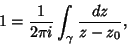(5)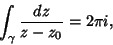(6)

and (3) becomes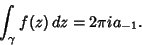(7)

The quantity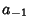is known as the Residue ofat. Generalizing to a curve passing through multiple poles, (7) becomes(8)

whereis the Winding Number and thesuperscript denotes the quantity corresponding to Pole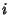.

If the path does not completely encircle the Residue, take the Cauchy Principal Value to obtain(9)

Ifhas only Isolated Singularities, then(10)

The residues may be found without explicitly expanding into a Laurent Series as follows:(11)

Ifhas a Pole of orderat, then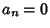for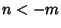and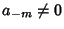. Therefore,(12)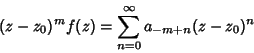(13)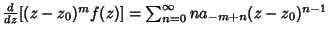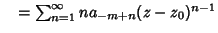(14)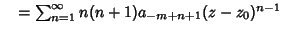(15)
Iterating,(16)
So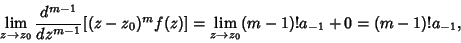(17)

and the Residue is(18)

This amazing theorem says that the value of a Contour Integral in the Complex Plane depends only on the properties of a few special points inside the contour.

See also Cauchy Integral Formula, Cauchy Integral Theorem, Contour Integral, Laurent Series, Pole, Residue (Complex Analysis)